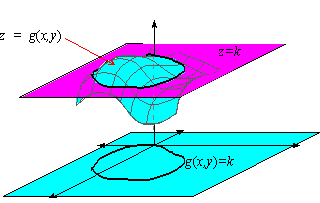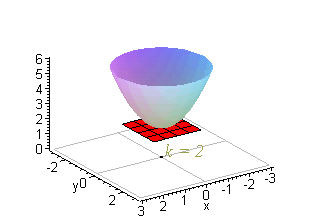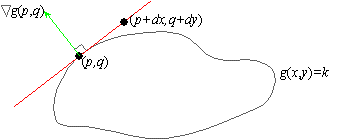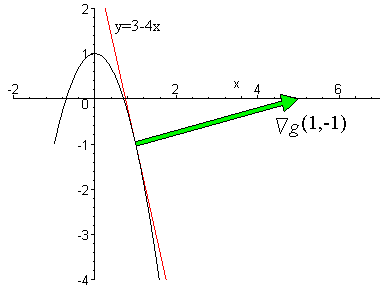### Gradients and Level Curves

In this section, we use the gradient and the chain rule to investigate horizontal and vertical slices of a surface of the form z = g( x,y) . To begin with, if k is constant, then g(x,y) = k is called the level curve of g( x,y) of level k and is the intersection of the horizontal plane z = k and the surface z = g( x,y) :In particular, g( x,y) = k is a curve in the xy-plane.

EXAMPLE 1    Construct the level curves of g( x,y) = x2+y2+2 for k = 2,3,4,5, and 6.

Solution: To begin with, g( x,y) = k is of the form x2+y2+2 = k, which simplifies to
 x2+y2 = k-2
If k = 2, then x2+y2 = 0 which is true only if ( x,y) = ( 0,0) . For k = 3,4,5,and 6, we have
 k = 3:
 x2+y2 = 1
 k = 5:
 x2+y2 = 3
 k = 4:
 x2+y2 = 2
 k = 6:
 x2+y2 = 4
These are circles centered at ( 0,0) with radii 1,ų2,ų3, and 2, respectively.If g( x,y) is differentiable, then the level curve g( x,y) = k can be parametrized by a vector-valued function of the form r( t) = á x( t) ,y(t) ñ . If v denotes the velocity of r, then the chain rule implies that
d dt
g( x,y) =  d dt
k       čg·v = 0
Thus, čg is perpendicular to the tangent line to g(x,y) = k at the point ( p,q) .We say that čg( p,q) is normal to the curve g( x,y) = k at ( p,q) .

EXAMPLE 2    Show that the gradient is normal to the curve y = 1-2x2 at the point ( 1,-1) .

Solution: To do so, we notice that 2x2+y = 1. Thus, the curve is of the form g( x,y) = 1 where g( x,y) = 2x2+y. The gradient of g is
 čg = á 4x,1 ñ
Thus, at ( 1,-1) , we have čg( 1,-1) = á 4,1 ñ . However, if y = 1-2x2, then y' = -4x, and when x = 1, we have y'( 1) = -4. That is, a run of 1 leads to a rise of -4, so that the slope corresponds to the vector
 m = á run,rise ñ = á1,-4 ñ
Clearly, čg·m = á 4,1 ñ · á 1,-4 ñ = 0, thus implying that čg is perpendicular to the tangent line to y = 1-2x2 at ( -1,1) .Check Your Reading: Are there any level curves of g( x,y) = x2+y2+2 with a level lower than k = 2?